# Kruskal-Wallis Test

This is a non-parametric version of the F-Test (ANOVA). The test statistic is: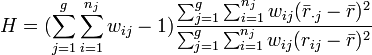$H = (\sum_{j=1}^g\sum_{i=1}^{n_j}w_{ij}-1)\frac{\sum_{j=1}^g\sum_{i=1}^{n_j} w_{ij} (\bar{r}_{\cdot j} - \bar{r})^2}{\sum_{j=1}^g\sum_{i=1}^{n_j} w_{ij} (r_{ij} - \bar{r})^2}$

where: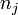$n_j$ is the number of observations in group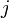$j$ of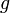$g$groups,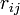$r_{ij}$ is the rank of the$i$th observation from group$j$ where the ranking is computed across all the groups with a 1 assigned to the lowest value and the average rank is used for ties,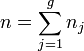$n = \sum^g_{j=1} n_j$,$w_{ij}$ is the Calibrated Weight,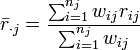$\bar{r}_{\cdot j} = \frac{\sum_{i=1}^{n_j}{w_{ij} r_{ij}}}{{\sum_{i=1}^{n_j}w_{ij}}}$,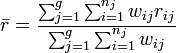$\bar{r} = \frac{\sum_{j=1}^g\sum_{i=1}^{n_j}w_{ij} r_{ij}}{\sum_{j=1}^g\sum_{i=1}^{n_j}w_{ij}}$,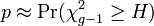$p\approx \Pr(\chi^2_{g-1} \ge H)$